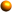Professor Freedman's Elementary Algebra SyllabusChapter 1 1.1 Sets of Numbers & the Real Number Line 1.2 Exponents, Square Roots, & the Order of Operations 1.3 Addition of Real Numbers 1.4 Subtraction of Real Numbers 1.5 Multiplication & Division of Real Numbers 1.6 Simplifying ExpressionsChapter 2 2.1 Addition, Subtraction, Multiplication & Division Properties of Equality 2.2 Solving Linear Equations 2.3 Clearing Fractions & Decimals in Equations 2.4 Applications of Linear Equatiions 2.6 Formulas & Applications of Geometry 2.7 Mixture Applications & Motion 2.8 Linear EqualitiesChapter 3 3.1 The Rectangular Coordinate System 3.2 Linear Equations in Two VariablesChapter 4 4.1 Solving Systems of Equations by Graphing 4.2  Solving Systems of Equations by Substitution 4.3 Solving Systems of Equations by Addition 4.4 Applications of Linear Equations in Two VariablesChapter 5 5.1 Exponents: Multiplying & Dividing Common Bases 5.2 More Properties of Exponents 5.3 Zero & Negative Exponents 5.5 Addition & Subtraction of Polynomials 5.6 Multiplication of Polynomials 5.7 Division of PolynomialsChapter 6 6.1 Greatest Common Factor & Factoring by Grouping 6.2 Factoring Trinomials of the Form x2+bx+c 6.3 Factoring Trinomials : Trial & Error Method 6.4 Factoring Trinomials AC Method 6.5 Difference of Squares 6.7 Solving Equations Using the Zero Product RuleChapter 7 7.1 Introduction to Rational Expressions 7.2 Multiplication & Division of Rational Expressions 7.3 Least Common Denominator 7.4 Addition & Subtraction of Rational Exprssions 7.6 Rational Equations 7.7 Applications of Rational Equatioons & ProportionsChapter 8 8.1 Introduction to Roots & Radicals 8.2 Simplifying Radicals 8.3 Addition & Subtraction of Radicals 8.4 Multiplication of RadicalsTHE FINAL EXAM WILL BE ADMINISTERED ON THE LAST DAY OF CLASS !!!! SCHOOL CLOSING NUMBER 559 Professor Ellen Freedman, Camden County College, 227-7200 Ext. 4712 email: discover@mathpower.com All Contents Copyright©1997-2017 by Ellen Freedman, All Rights Reserved.